# Density Functional Theory

01/01/1965View on timeline

Density functional theory (DFT) is a computational quantum mechanical modelling method used in physics, chemistry and materials science to investigate the electronic structure (principally the ground state) of many-body systems, in particular atoms, molecules, and the condensed phases. Using this theory, the properties of a many-electron system can be determined by using functionals, i.e. functions of another function, which in this case is the spatially dependent electron density. Hence the name density functional theory comes from the use of functionals of the electron density. DFT is among the most popular and versatile methods available in condensed-matter physics, computational physics, and computational chemistry.

DFT has been very popular for calculations in solid-state physics since the 1970s. However, DFT was not considered accurate enough for calculations in quantum chemistry until the 1990s, when the approximations used in the theory were greatly refined to better model the exchange and correlation interactions. Computational costs are relatively low when compared to traditional methods, such as exchange only Hartree–Fock theory and its descendants that include electron correlation.

Despite recent improvements, there are still difficulties in using density functional theory to properly describe: intermolecular interactions (of critical importance to understanding chemical reactions), especially van der Waals forces (dispersion); charge transfer excitations; transition states, global potential energy surfaces, dopant interactions and some strongly correlated systems; and in calculations of the band gap and ferromagnetism in semiconductors. The incomplete treatment of dispersion can adversely affect the accuracy of DFT (at least when used alone and uncorrected) in the treatment of systems which are dominated by dispersion (e.g. interacting noble gas atoms) or where dispersion competes significantly with other effects (e.g. in biomolecules). The development of new DFT methods designed to overcome this problem, by alterations to the functional or by the inclusion of additive terms, is a current research topic.

n the context of computational materials science, ab initio (from first principles) DFT calculations allow the prediction and calculation of material behaviour on the basis of quantum mechanical considerations, without requiring higher order parameters such as fundamental material properties. In contemporary DFT techniques the electronic structure is evaluated using a potential acting on the system’s electrons. This DFT potential is constructed as the sum of external potentials Vext, which is determined solely by the structure and the elemental composition of the system, and an effective potential Veff, which represents interelectronic interactions. Thus, a problem for a representative supercell of a material with n electrons can be studied as a set of n one-electron Schrödinger-like equations, which are also known as Kohn–Sham equations.

Although density functional theory has its roots in the Thomas–Fermi model for the electronic structure of materials, DFT was first put on a firm theoretical footing by Walter Kohn and Pierre Hohenberg in the framework of the two Hohenberg–Kohn theorems (H–K). The original H–K theorems held only for non-degenerate ground states in the absence of a magnetic field, although they have since been generalized to encompass these.

The first H–K theorem demonstrates that the ground state properties of a many-electron system are uniquely determined by an electron density that depends on only three spatial coordinates. It set down the groundwork for reducing the many-body problem of N electrons with 3N spatial coordinates to three spatial coordinates, through the use of functionals of the electron density. This theorem has since been extended to the time-dependent domain to develop time-dependent density functional theory (TDDFT), which can be used to describe excited states.

The second H–K theorem defines an energy functional for the system and proves that the correct ground state electron density minimizes this energy functional.

In work that later won them the Nobel prize in chemistry, The H–K theorem was further developed by Walter Kohn and Lu Jeu Sham to produce Kohn–Sham DFT (KS DFT). Within this framework, the intractable many-body problem of interacting electrons in a static external potential is reduced to a tractable problem of noninteracting electrons moving in an effective potential. The effective potential includes the external potential and the effects of the Coulomb interactions between the electrons, e.g., the exchange and correlation interactions. Modeling the latter two interactions becomes the difficulty within KS DFT. The simplest approximation is the local-density approximation (LDA), which is based upon exact exchange energy for a uniform electron gas, which can be obtained from the Thomas–Fermi model, and from fits to the correlation energy for a uniform electron gas. Non-interacting systems are relatively easy to solve as the wavefunction can be represented as a Slater determinant of orbitals. Further, the kinetic energy functional of such a system is known exactly. The exchange–correlation part of the total energy functional remains unknown and must be approximated.

Another approach, less popular than KS DFT but arguably more closely related to the spirit of the original H–K theorems, is orbital-free density functional theory (OFDFT), in which approximate functionals are also used for the kinetic energy of the noninteracting system.

#### Application

In general, density functional theory finds increasingly broad application in the chemical and materials sciences for the interpretation and prediction of complex system behavior at an atomic scale. Specifically, DFT computational methods are applied for the study of systems to synthesis and processing parameters. In such systems, experimental studies are often encumbered by inconsistent results and non-equilibrium conditions. Examples of contemporary DFT applications include studying the effects of dopants on phase transformation behavior in oxides, magnetic behaviour in dilute magnetic semiconductor materials and the study of magnetic and electronic behavior in ferroelectrics and dilute magnetic semiconductors. Also, it has been shown that DFT has a good results in the prediction of sensitivity of some nanostructures to environment pollutants like sulfur dioxide or acrolein as well as prediction of mechanical properties.

In practice, Kohn–Sham theory can be applied in several distinct ways depending on what is being investigated. In solid state calculations, the local density approximations are still commonly used along with plane wave basis sets, as an electron gas approach is more appropriate for electrons delocalised through an infinite solid. In molecular calculations, however, more sophisticated functionals are needed, and a huge variety of exchange–correlation functionals have been developed for chemical applications. Some of these are inconsistent with the uniform electron gas approximation, however, they must reduce to LDA in the electron gas limit. Among physicists, probably the most widely used functional is the revised Perdew–Burke–Ernzerhof exchange model (a direct generalized gradient parametrization of the free electron gas with no free parameters); however, this is not sufficiently calorimetrically accurate for gas-phase molecular calculations. In the chemistry community, one popular functional is known as BLYP (from the name Becke for the exchange part and Lee, Yang and Parr for the correlation part). Even more widely used is B3LYP which is a hybrid functional in which the exchange energy, in this case from Becke's exchange functional, is combined with the exact energy from Hartree–Fock theory. Along with the component exchange and correlation funсtionals, three parameters define the hybrid functional, specifying how much of the exact exchange is mixed in. The adjustable parameters in hybrid functionals are generally fitted to a 'training set' of molecules. Unfortunately, although the results obtained with these functionals are usually sufficiently accurate for most applications, there is no systematic way of improving them (in contrast to some of the traditional wavefunction-based methods like configuration interaction or coupled cluster theory). Hence in the current DFT approach it is not possible to estimate the error of the calculations without comparing them to other methods or experiments.C-60 with isosurface of ground-state electron density as calculated with DFT.

DFT is supported by many quantum chemistry and solid state physics software packages, often along with other methods.

Density Functional Theory of Electronic Structure.pdf

Density Functional Theory - Derivation and formalism

REFERENCES

Durham University. Available in: http://cmt.dur.ac.uk/sjc/thesis_prt/node24.html. Access in: 20/10/2018.

Wikipedia. Available in: https://en.wikipedia.org/wiki/Density_functional_theory. Access in: 20/10/2018.

Comment

Author

Info

Published in 21/10/2018

Updated in 19/02/2021

All events in the topic Condensed Matter Physics:

Invalid Date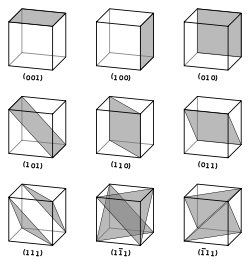Classification of crystalline symmetries
Invalid Date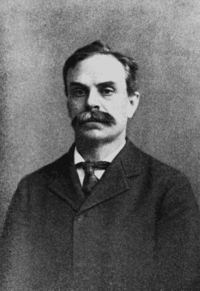Hall Effect
Invalid Date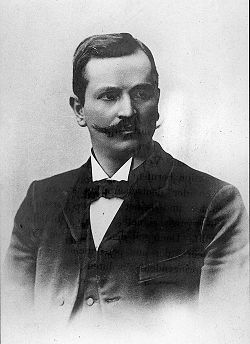Drude and Lorentz model on electric conduction
Invalid Date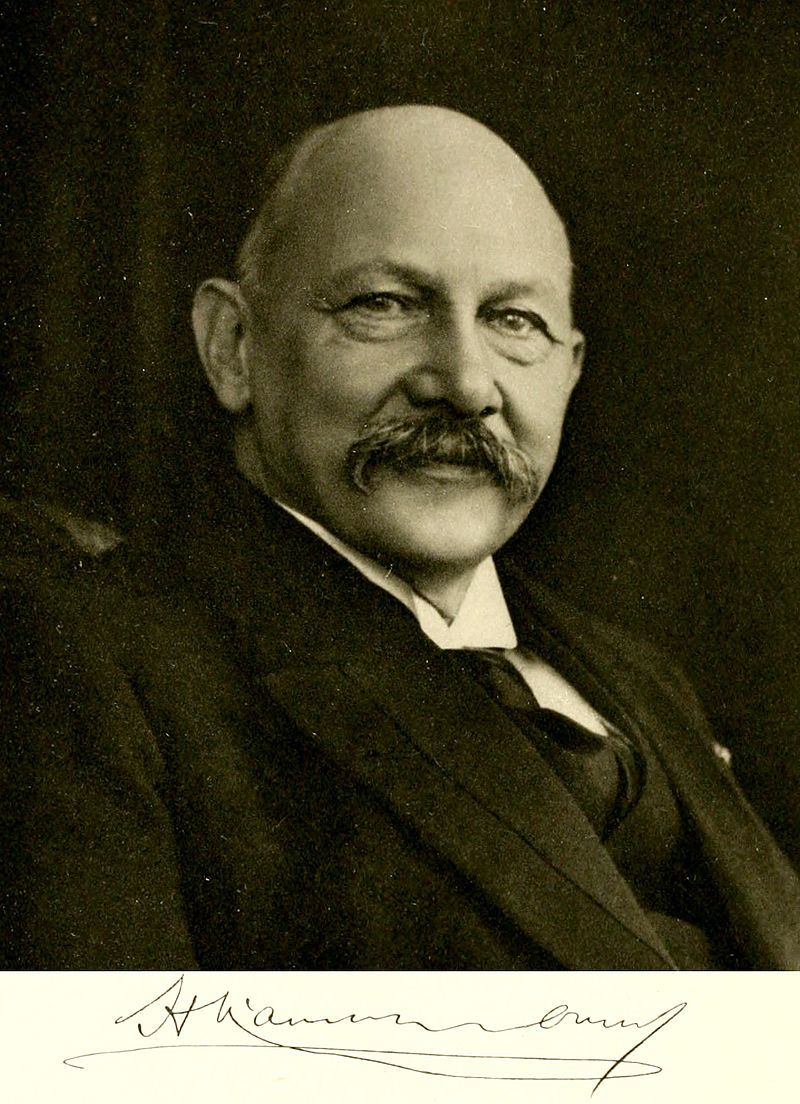Discovery of mercury superconductivity by Onnes
Invalid DateH. K. Onnes receives the Nobel Prize
Invalid DateMax von Laue receives the Nobel Prize
Invalid Date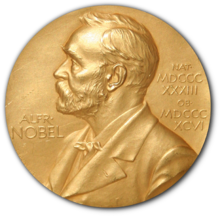Sir W. H. Bragg and W. L. Bragg share Nobel Prize
Invalid DateSir C. V. Raman receives the Nobel Prize
Invalid Date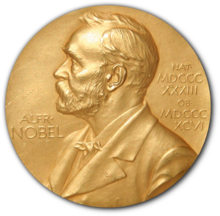Shockley, Bardeen and Brattain share Nobel Prize
Invalid Date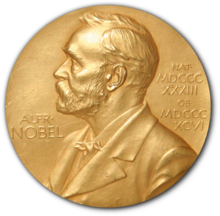L. D. Landau receives the Nobel Prize
Invalid Date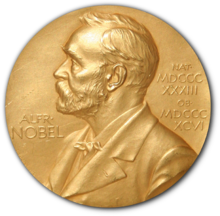Bardeen, Cooper and Schrieffer share the Nobel Prize
Invalid DateStudy of Crystals using X-rays by W.H. & W.L. Bragg
Invalid DateDiscovery of X-ray diffraction by Crystals by Van Laue
Invalid Date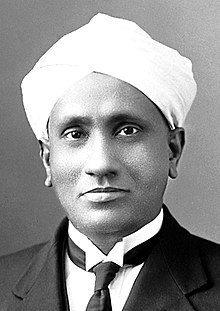Raman scattering
Invalid Date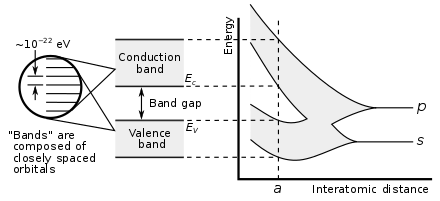Quantum Theory in Solids
Invalid Date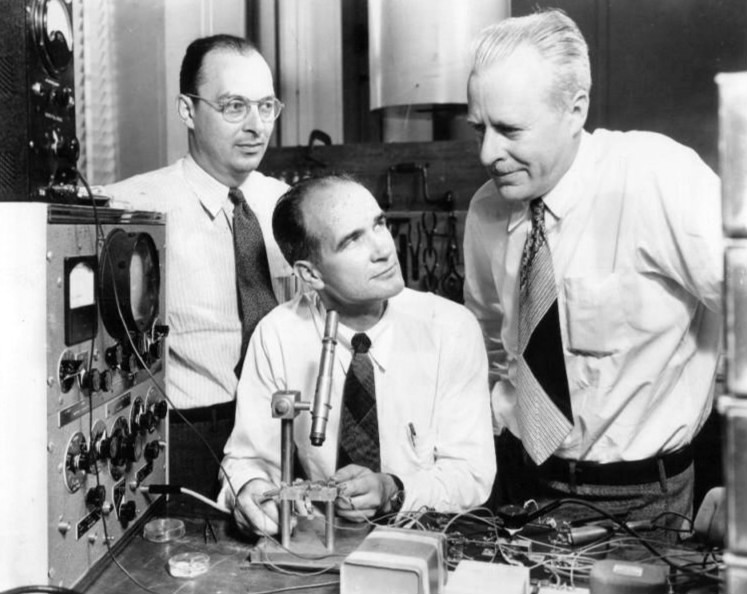Transistor Effect
Invalid DateThe superconductivity theory of Ginzburg-Landau
Invalid Date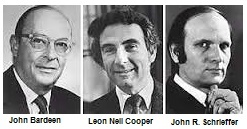Theory of Superconductivity BCS
Invalid Date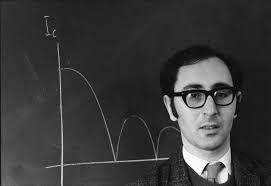Josephson Effect tunneling in superconductors
Invalid DateDensity Functional Theory
Invalid DateSuperfluid helium-3
Invalid DateLiquid Crystal Theory
Invalid Date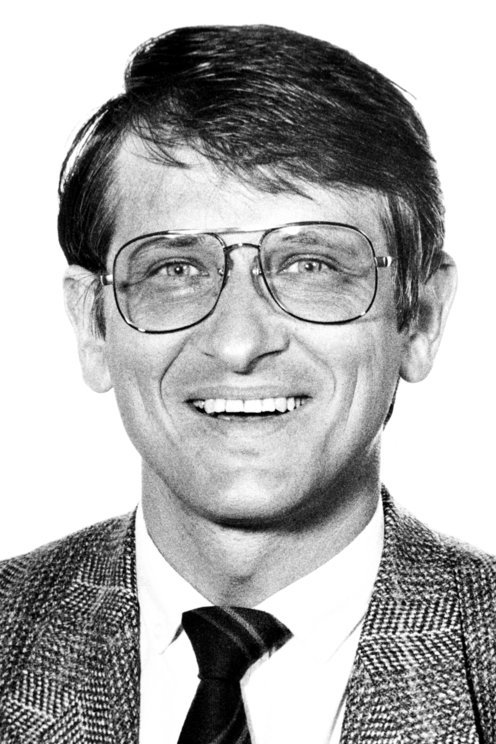Integer and Fractional Quantum Hall Effect
Invalid Date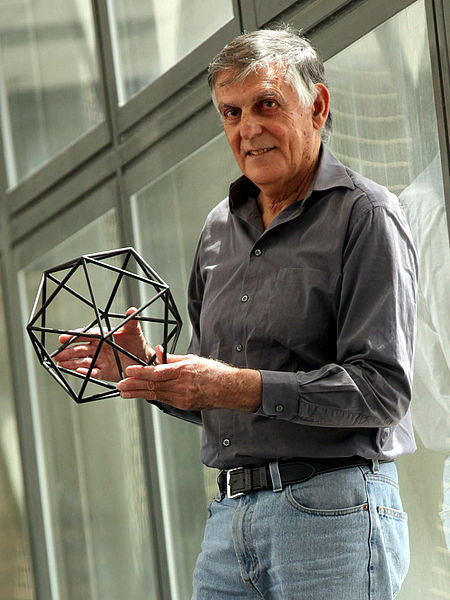Discovery of Quasi-crystals
Invalid DateFullerene 60
Invalid Date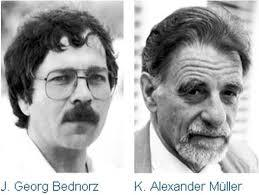High-temperature superconductivity
Invalid Date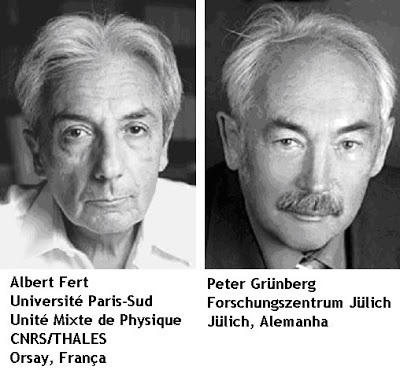Giant magnetoresistance
Invalid Date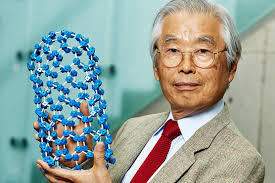Carbon nanotube
Invalid Date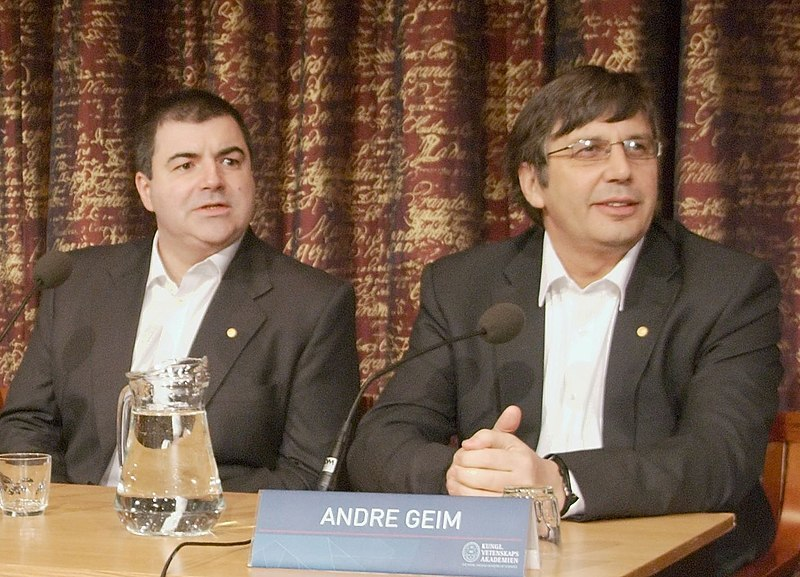Discovering Graphene
Invalid DateExcitonium